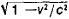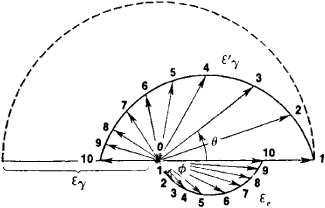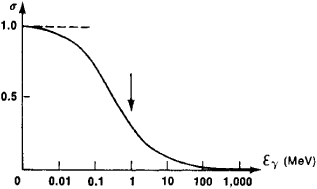# Compton effect

Also found in: Dictionary, Medical, Wikipedia.

## Compton effect

[for A. H. ComptonCompton, Arthur Holly,
1892–1962, American physicist, b. Wooster, Ohio, grad. College of Wooster (B.S., 1913), Ph.D. Princeton, 1916. He was professor and head of the department of physics at Washington Univ., St. Louis (1920–23), and professor of physics at the Univ.
], increase in the wavelengths of X rays and gamma rays when they collide with and are scattered from loosely bound electrons in matter. This effect provides strong verification of the quantum theory since the theoretical explanation of the effect requires that one treat the X rays and gamma rays as particles or photons (quanta of energy) rather than as waves. The classical treatment of these rays as waves would predict no such effect. According to the quantum theory a photon can transfer part of its energy and linear momentum to a loosely bound electron in a collision. Since the energy and magnitude of linear momentum of a photon are proportional to its frequency, after the collision the photon has a lower frequency and thus a longer wavelength. The increase in the wavelength does not depend upon the wavelength of the incident rays or upon the target material. It depends only upon the angle that is formed between the incident and scattered rays. A larger scattering angle will yield a larger increase in wavelength. The effect was discovered in 1923. It is used in the study of electrons in matter and in the production of variable energy gamma-ray beams.

## Compton effect

The increase in wavelength of electromagnetic radiation, observed mainly in the x-ray and gamma-ray region, on being scattered by material objects. This increase in wavelength is caused by the interaction of the radiation with the weakly bound electrons in the matter in which the scattering takes place. The Compton effect illustrates one of the most fundamental interactions between radiation and matter and displays in a very graphic way the true quantum nature of electromagnetic radiation. Together with the laws of atomic spectra, the photoelectric effect, and pair production, the Compton effect has provided the experimental basis for the quantum theory of electromagnetic radiation. See Angular momentum, Atomic structure and spectra, Electron-positron pair production, Light, Photoemission, Quantum mechanics, Uncertainty principle

Perhaps the greatest significance of the Compton effect is that it demonstrates directly and clearly that in addition to its wave nature with transverse oscillations, electromagnetic radiation has a particle nature and that these particles, the photons, behave quite like material particles in collisions with electrons. This discovery by A. H. Compton and P. Debye led to the formulation of quantum mechanics by W. Heisenberg and E. Schrödinger and provided the basis for the beginning of the theory of quantum electrodynamics, the theory of the interactions of electrons with the electromagnetic field.

The Compton effect has played a significant role in several diverse scientific areas. Compton scattering (often referred to as incoherent scattering, in contrast to Thomson scattering or also Rayleigh scattering, which are called coherent scattering) is important in nuclear engineering (radiation shielding), experimental and theoretical nuclear physics, atomic physics, plasma physics, x-ray crystalloghaphy, elementary particle physics, and astrophysics, to mention some of these areas. In addition the Compton effect provides an important research tool in some branches of medicine, in molecular chemistry and solid-state physics, and in the use of high-energy electron accelerators and charged-particle storage rings.

The development of high-resolution silicon and germanium semiconductor radiation detectors opened new areas for applications of Compton scattering. Semiconductor detectors make it possible to measure the separate probabilities for Rayleigh and Compton scattering. An effective atomic number has been assigned to compounds that appears to successfully correlate theory with Rayleigh-Compton ratios.

Average density can be measured by moving to higher energies where Compton scattering does not have to compete with Rayleigh scattering. At these energies, Compton scattering intensity has been successfully correlated with mass density. An appropriate application is the measurement of lung density in living organisms.

The ability to put large detectors in orbit above the Earth' atmosphere has created the field of gamma-ray astronomy. This field is now based largely on the data from the Compton Gamma-Ray Observatory, all of whose detectors made use of the Compton effect (although not exclusively). See Gamma-ray detectors, Gamma rays, X-rays

## Compton Effect

the elastic scattering of electromagnetic radiation by free electrons, accompanied by an increase in wavelength; it is observed during scattering of radiation of short wavelength—X rays and gamma rays. The corpuscular properties of radiation were fully revealed for the first time in the Compton effect.

The Compton effect was discovered in 1922 by the American physicist A. Compton, who observed that X rays scattered in paraffin have a longer wavelength than the incident rays. Such a shift in wavelength could not be explained by classical theory. In fact, according to classical electrodynamics, under the influence of the periodic electric field of an electromagnetic (light) wave, an electron should oscillate with a frequency equal to that of the wave and consequently should radiate secondary (scattered) waves of the same frequency. Thus, in “classical” scattering (the theory of which was provided by the British physicist J. J. Thomson and is therefore called Thomson scattering) the wavelength of the light does not change.

An elementary theory of the Compton effect based on quantum concepts was given by Compton and independently by P. Debye. According to quantum theory a light wave is a stream of light quanta, or photons. Each photon has a definite energy ع = hv = hc/λ and a definite momentum pγ = (h/λ)n, where λ is the wavelength of the incident light (v is its frequency), c is the speed of light, h is Planck’s constant, and n is the unit vector in the direction of propagation of the wave (the subscript γ denotes a photon). In quantum theory the Compton effect appears as an elastic collision between two particles, the incident photon and the stationary electron. In every such collision event the laws of conservation of energy and momentum are obeyed. A photon that has collided with an electron transfers part of its energy and momentum to the electron and changes its direction of motion (it is scattered); the decrease in the photon’s energy signifies an increase in the wavelength of the scattered light. The electron, which previously had been stationary, receives energy and momentum from the photon and is set in motion (it experiences recoil). The direction of motion of the particles after the collision, as well as their energy, is determined by the laws of conservation of energy and momentum (Figure 1).Figure 1.

Note to Figure 1. Elastic collision of a photon and an electron in the Compton effect. Before the collision the electron was stationary: pγ and p’γ are the momentum of the incident and scattered photons, pe = mvis the momentum of the recoil electron (v is its velocity), ( is the photon’s scattering angle, and ø is the angle of escape of the recoil electron relative to the direction of the incident photon.

Simultaneous solution of the equations expressing the equality of the summed energies and momentums of the particles before and after the collision (assuming that the electron is stationary before the collision) gives Compton’s formula for the shift Δλ in the wavelength of the light:

Δλ = λ’ − λ = λ0(1 ˗ cos θ)

Here λ’ is the wavelength of the scattered light, θ is the photon’s scattering angle, and λ0 = h/mc = 2.426 × 10˗10 cm = 0.024 angstrom (Å) is the “Compton wavelength” of the electron (m is the mass of the electron). It follows from Compton’s formula that the shift Δλ in the wavelength does not depend on the wavelength λ of the incident light itself. It is solely determined by the scattering angle θ of the photon and is maximal when θ = 180°, that is, when scattering is straight back: Δλmax = 2λo.

Expressions for the energy عe of the recoil, or “Compton,” electron as a function of the angle ø of its escape may be obtained from the same equations. The dependence of the energy ع’ γ of the scattered photon on the scattering angle θ, as well as the dependence of عe on ø, which is related to it, is shown in Figure 2. From the figure it is apparent that the recoil electrons always have a velocity component in the direction of motion of the incident photon (that is, ø does not exceed 90°).

Experiment has confirmed all the above theoretical predictions. The correctness of the corpuscular concepts of the mechanism of the Compton effect—and thus the correctness of the basic assumptions of quantum theory—has been experimentally proved.

In actual experiments on the scattering of photons by matter, the electrons are not free but are bound to atoms. If the energy of the photons is high in comparison with the binding energy of the electrons in the atom (X-ray and gamma-ray photons), then the electrons experience a recoil strong enough to expel them from the atom. In this case the photon scattering proceeds as if with free electrons. However, if the energy of the photon is not sufficient to tear the electron from the atom, then the photon exchanges energy and momentum with the entire atom. Since the mass of the atom is very great compared to the photon’s equivalent mass (which, according to the theory of relativity, equals £y/c2), the recoil is virtually nonexistent; therefore, the photonFigure 2. Dependence of the energy ع’λ of the scattered photon on the scattering angle θ (for convenience, only the upper half of the symmetrical curve is depicted) and the dependence of the energy عe of the recoil electron on the angle of escape 0 (lower half of the curve). Quantities related to the same collision event are labeled with identical numbers. The vectors drawn from point 0, at which the collision between the proton with energy عγ and the stationary electron occurred, to corresponding points on the curves depict the state of the particle after scattering: the magnitudes of the vectors give the energy of the particles, and the angles formed by the vectors with the direction of the incident photon define the scattering angle ø and the angle 0 of the recoil electron’s path. (The graph was plotted for the case of scattering of “hard” X rays with wavelength hc/عγ = γo = 0.024 Å.)

is scattered without a change in its energy (that is, without a change in its wavelength, or “coherently”). In heavy atoms only the peripheral electrons are weakly bound (in contrast to the electrons filling the inner shells of the atom), and therefore the spectrum of the scattered radiation has both a shifted (Compton) line, from scattering by the peripheral electrons, and an un-shifted (coherent) line, from scattering by the entire atom. With increasing atomic number (nuclear charge) the electron binding energy increases, the relative intensity of the Compton line decreases, and that of the coherent line increases.

The motion of the electrons in atoms leads to a broadening of the Compton lines in the scattered radiation. This occurs because the wavelength of the incident light appears to be slightly changed for moving electrons; in addition, the amount of change depends on the magnitude and direction of the electron’s velocity (the Doppler effect). Careful measurements of the intensity distribution in a Compton line, which reflects the velocity distribution of the electrons in the material, has confirmed the correctness of quantum theory, according to which electrons obey Fermi-Dirac statistics.

The simplified theory of the Compton effect examined here does not permit the calculation of all characteristics of Compton scattering, particularly the intensity of photon scattering at various angles. A complete theory of the Compton effect is provided by quantum electrodynamics. The intensity of Compton scattering depends on both the scattering angle and the wavelength of the incident radiation. Asymmetry is observed in the angular distribution of the scattered photons: more photons are scattered forward, and the asymmetry increases with increasing energy of the incident photons. The total intensity of Compton scattering decreases with an increase in the energy of the primary photons (Figure 3); this indicates that the probability of the Compton scattering of a photon passing through matter diminishes with decreasing energy. Such a dependence of intensity on £y determines the place of Compton scattering among the other effects of interaction between matter and radiation that are responsible for loss of energy by photons in their passage through matter. For example, in lead the Compton effect makes the main contribution to the energy loss of photons at energies of the order of 1–10 mega electron volts, or MeV (in a lighter element, aluminum, this range is 0.1–30.0 MeV); below this region it is surpassed by the photoelectric effect, and above it by pair production.

Compton scattering is used extensively in studying the gamma radiation of nuclei; it is also the basis of the principle of operation of some gamma spectrometers.

The Compton effect is possible not only for electrons but also for other charged particles, such as protons; however, because of the proton’s large mass its recoil is noticeable only during the scattering of photons with very high energy.

The double Compton effect consists of the formation of two scattered photons in place of a single incident photon during scattering by a free electron. The existence of this process follows from quantum electrodynamics; it was first observed in 1952. Its probability is approximately a hundred times less than that of the ordinary Compton effect.Figure 3. Graph showing the dependence of the total Compton scattering intensity <r on the energy of the photon £y (in units of the total intensity of classical scattering); the arrow indicates the energy at which the production of electron-positron pairs begins

Inverse Compton effect. If the electrons on which electromagnetic radiation is scattered are relativistic (that is, if they are moving with speeds close to the speed of light), then in an elastic collision the wavelength of the radiation will decrease: the energy and momentum of the photons will increase at the expense of the energy and momentum of the electrons. This phenomenon is called the inverse Compton effect and is often used to explain the radiation mechanism of cosmic X-ray sources, the production of the X-ray component of the background galactic radiation, and the transformation of plasma waves into high-frequency electromagnetic waves.

### REFERENCES

Born, M. Atomnaia fizika, 3rd ed. Moscow, 1970. (Translated from English.)
Heitler, W. Kvantovaia teoriia izlucheniia. Moscow, 1956. (Translated from English.)
V. P. PAVLOV

## Compton effect

[′käm·tən i′fekt]
(quantum mechanics)
The increase in wavelength of electromagnetic radiation in the x-ray and gamma-ray region on being scattered by material objects; the scattering is due to the interaction of the photons with electrons that are effectively free. Also known as Compton-Debye effect.
Site: Follow: Share:
Open / Close